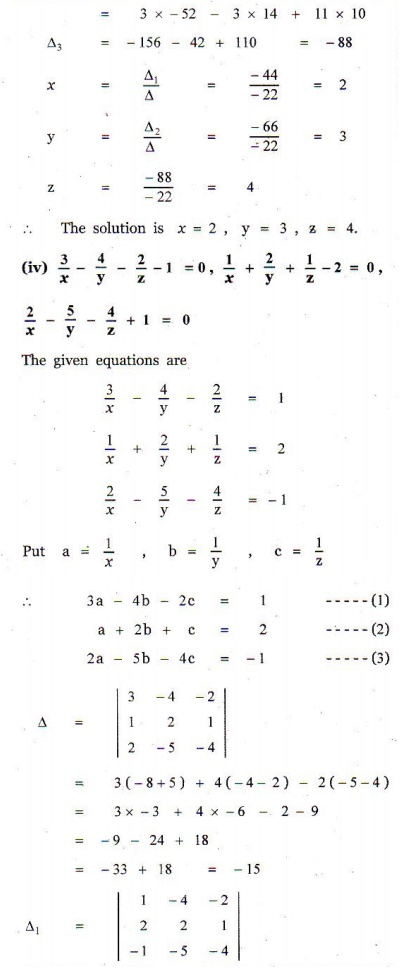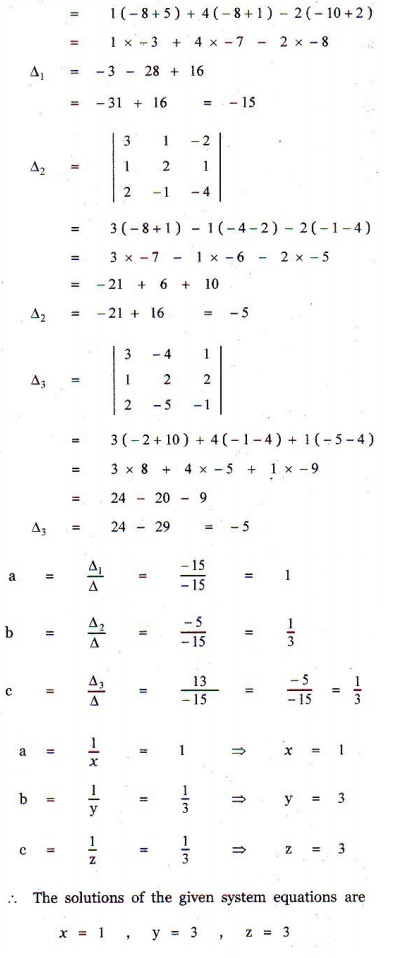Home | | Maths 12th Std | Exercise 1.4: Matrices: CramerŌĆÖs Rule

# Exercise 1.4: Matrices: CramerŌĆÖs Rule

Maths Book back answers and solution for Exercise questions - Solve the following systems of linear equations by CramerŌĆÖs rule

EXERCISE 1.4

1. Solve the following systems of linear equations by CramerŌĆÖs rule:

(i) 5x - 2 y +16 = 0, x + 3y - 7 = 02. In a competitive examination, one mark is awarded for every correct answer while ┬╝ mark is deducted for every wrong answer. A student answered 100 questions and got 80 marks. How many questions did he answer correctly ? (Use CramerŌĆÖs rule to solve the problem).3. A chemist has one solution which is 50% acid and another solution which is 25% acid. How much each should be mixed to make 10 litres of a 40% acid solution ? (Use CramerŌĆÖs rule to solve the problem).4. A fish tank can be filled in 10 minutes using both pumps A and B simultaneously. However, pump B can pump water in or out at the same rate. If pump B is inadvertently run in reverse, then the tank will be filled in 30 minutes. How long would it take each pump to fill the tank by itself ? (Use CramerŌĆÖs rule to solve the problem).5. A family of 3 people went out for dinner in a restaurant. The cost of two dosai, three idlies and two vadais is Ōé╣ 150. The cost of the two dosai, two idlies and four vadais is Ōé╣ 200. The cost of five dosai, four idlies and two vadais is Ōé╣ 250. The family has Ōé╣ 350 in hand and they ate 3 dosai and six idlies and six vadais. Will they be able to manage to pay the bill within the amount they had ?1. (i) x = -2, y = 3

(ii) x = 1/2 , y = 3

(iii) x = 2, y = 3, z = 4

(iv) x = 1, y = 3, z = 3

2. 84

3. 50% acid is 6 litres, 25% acid is 4 litres

4. Pump A : 15 minutes, Pump B : 30 minutes

5. Ōé╣ 30/-, Ōé╣ 10/-, Ōé╣ 30/-, yes

Tags : Problem Questions with Answer, Solution , 12th Mathematics : UNIT 1 : Applications of Matrices and Determinants
Study Material, Lecturing Notes, Assignment, Reference, Wiki description explanation, brief detail
12th Mathematics : UNIT 1 : Applications of Matrices and Determinants : Exercise 1.4: Matrices: CramerŌĆÖs Rule | Problem Questions with Answer, Solution